# SSC CGL EXAMS 2019 | Reasoning Ability Practice Questions (Day-20)

Dear Aspirants, Here we have given the Important SSC Exam 2019 Practice Test Papers. Candidates those who are preparing for SSC 2019 can practice these questions to get more confidence to Crack SSC 2019 Examination.

[WpProQuiz 4822]

1) In the following question, select the related letters from the given responses

MZE, NAJ, OBO, PCT, ?

a) QDY

b) QEZ

c) REA

d) N.O.T

2) In the following question, select the related letters from the given responses

A 6 2 5 B 5 7 6 C 5 2 9 D 4 8 4………….?

a) E444

b) E434

c) E289

d) E441

3) Find the odd number/ letters from the given alternatives.

a) (125, 225)

b) (221, 16)

c) (283, 900)

d) (226, 100)

4) Find the odd number/ letters from the given alternatives.

a) Metal

b) Non-Metal

c) Metalloid

d) Iron

5) If ‘Pen’ means ‘+’ and Pencil means ‘–‘, copy means ‘ ÷’, Eraser means ‘×’. Then find the value of the given Expression?

3 Pen 6 copy 8 Eraser 16 Pencil 4?

a) 15

b) 13

c) 11

d) 12

6) In each of the following question below are given some statements followed by some conclusions. Taking the given statements to be true even if they seem to be at variance from commonly known facts, read all the conclusions and then decide which of the given conclusion logically follows the given statements.

Statement:

I. All faculty are Expert.

II. All experts are Active.

III. No active is Brilliant.

Conclusion:

I. All faculty are Active.

II. No Expert is Brilliant.

III. Same Active is faculty.

a) Only I follow.

b) Only II follow

c) Only II & III follows.

d) All I, II & III follows.

7) Find the shortest distance between point A to D to the given figure?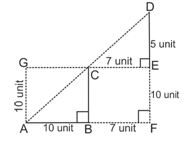a) √514 unit

b) √541

c) √415

d) None of t hese

Shortest Route is A to D directly for the hypotenuse of AFD, here AD is hypotenuse, and D F is perpendicular

AF is base.

8) Which answer figure will complete the question figure?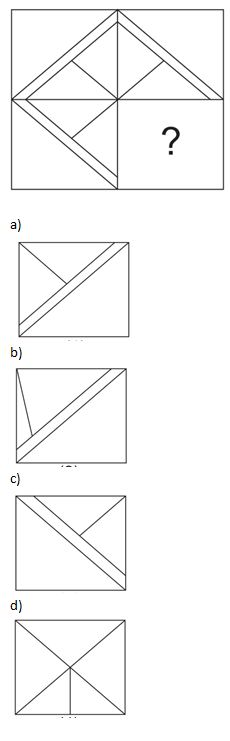9) What should come in place of fill in the blanks?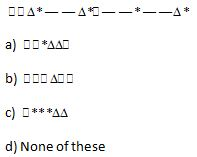10) A man said to a lady “your Son is my only Son” than how man is related to that lady?

a) Man is husband of lady

b) Man and women is spouse

c) Lady is wife of Man

d) All True.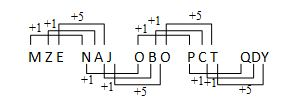A = (26 – 1)2 = 625

B = (26 – 3)2 = 529

C = (26 – 2)2 = 576

D = (26 – 4)2 = 484

So, E = (26 – 5)2 = 441.

125 => (1+2) × 5 = 152 = 225

221 => (2+2) × 1 = 42 = 16

283 => (2+8) × 3 = 10 ×3 = 302 = 900

So, 226 => (2 + 2) × 6 = 242 = 576 ≠100

‘Iron’ is an example of ‘Metal’. But all the other three options ‘Metal’, ‘Non-Metal’ and ‘Metalloid’ are types of element so Iron is odd.

3 + 6 ÷ 8 × 16 – 4

= 3 + (6/8) × 16 – 4

= 15 – 4

= 11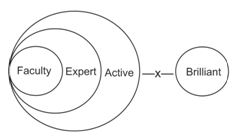Shortest Route is A to D directly for the hypotenuse of AFD, here AD is hypotenuse, and D F is perpendicular

AF is base.

Now,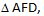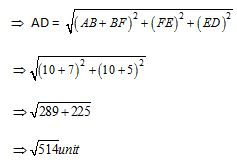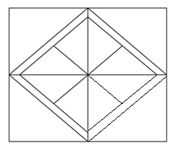Series is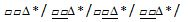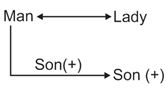*********************************

SSC CGL EXAMS 2018 | Reasoning Ability Practice Questions  (Day-19)

SSC CGL EXAMS 2018 | Reasoning Ability Practice Questions  (Day-18)

SSC CGL EXAMS 2018 | Reasoning Ability Practice Questions  (Day-17)